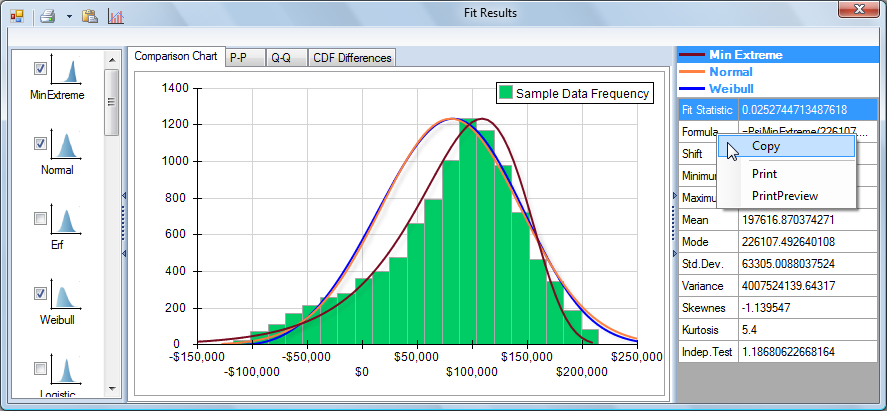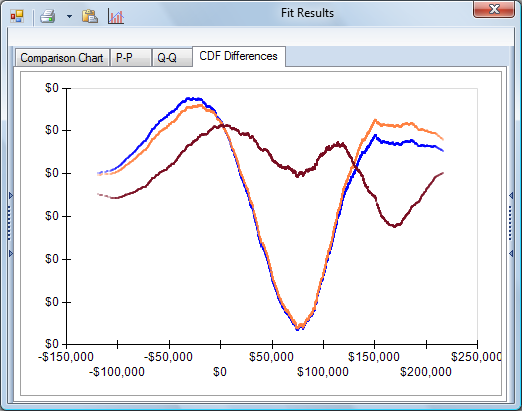## Automatically Fit Distributions and Parameters to Samples

Risk Solver can automatically fit a wide range of analytic probability distributions to user-supplied data for an uncertain variable, or to simulation results for an uncertain function. Nearly 30 continuous and discrete distributions can be fitted, with automatic shifting of the distribution center and optimization of distribution parameters. You can display overlay charts, P-P and Q-Q charts, and CDF Difference charts to help you choose the most appropriate fit.

To fit a distribution to data in your spreadsheet, simply select the data and click the Fit icon on the Risk Solver Platform ribbon.  Alternatively, to fit a distribution for an uncertain variable or function, click the Fit icon in the associated Uncertain Variable or Uncertain Function dialog. This displays the Fit Options dialog as shown below:For Continuous data, you can choose to rank the fitted distributions by the Chi Square, Kolmogorov-Smirnoff, or Anderson-Darling goodness-of-fit statistic. For Discrete data, only the Chi Square statistic is meaningful (and may be selected).  Risk Solver computes and displays a ranked list of candidate fitted distributions. Initially, the distribution with the best fit statistic is shown in an overlay chart with the sample data.You can select any distribution in the left pane to display its PDF in the center chart area, and show its fitted parameters, analytic moments, and goodness-of-fit statistic in the right pane. As shown below, you can select more than one distribution in the left pane. PDFs for the selected distributions will appear as overlays on the chart of sample data from the simulation. A list of the selected distributions will appear at the top of the right pane; when you select one of these, its fitted parameters, analytic moments, and goodness-of-fit statistic will be displayed.The second cell in the right pane contains a PSI Distribution function call with fitted parameters. You can right-click this cell and select Copy as shown, then paste the formula string into a cell or any other document.

To help you choose the most appropriate distribution, you can click the tabs in this dialog to display a P-P chart, Q-Q chart, or CDF Difference chart.A P-P (Probability-Probability) chart plots the CDF (Cumulative Distribution Function) of the fitted probability distribution against the cumulative frequency of the sample data. A perfectly fitted distribution will plot as a straight line from the lower left to the upper right edge of the chart.A Q-Q (Quantile-Quantile) chart plots the quantiles (similar to percentiles) of the fitted probability distribution against the quantiles of the sample data. Again, a perfectly fitted distribution will plot as a straight line from the lower left to the upper right edge of the chart.A CDF Difference chart plots the difference, at each point, between the CDF of the fitted distribution and the cumulative frequency of the sample data. A perfectly fitted distribution will plot as a 'flat' straight line coinciding with the x-axis.

When you dismiss the Fit Results dialog,  Risk Solver gives you the option of placing the identifed PSI distribution function in a cell on your worksheet (if you are fitting user-supplied data in your worksheet) or drawing the PDF of the distribution you select in the "host" dialog (if you are fitting an uncertain variable or function).  In the Uncertain Variable dialog, the fitted distribution becomes the new distribution for this uncertain variable.  In the Uncertain Function dialog, the fitted distribution PDF is overlaid on the Frequency, Cumulative Frequency, and Reverse Cumulative Frequency chart.

< Back to: Choosing Distributions